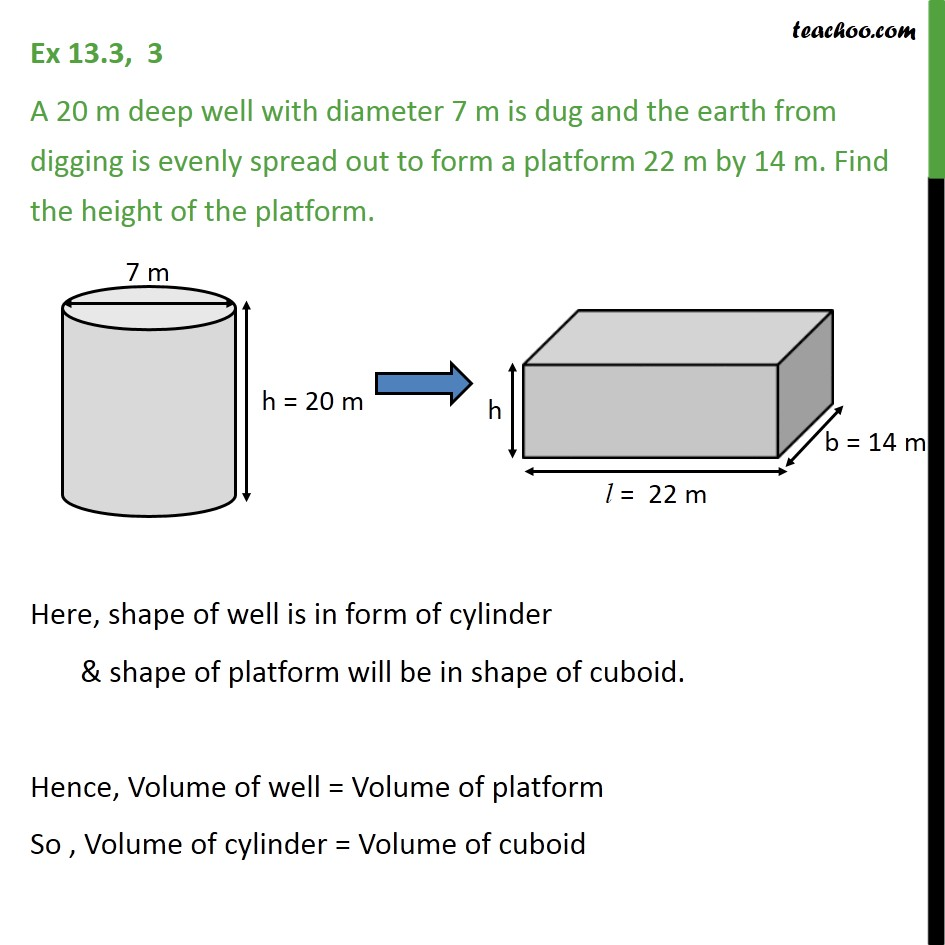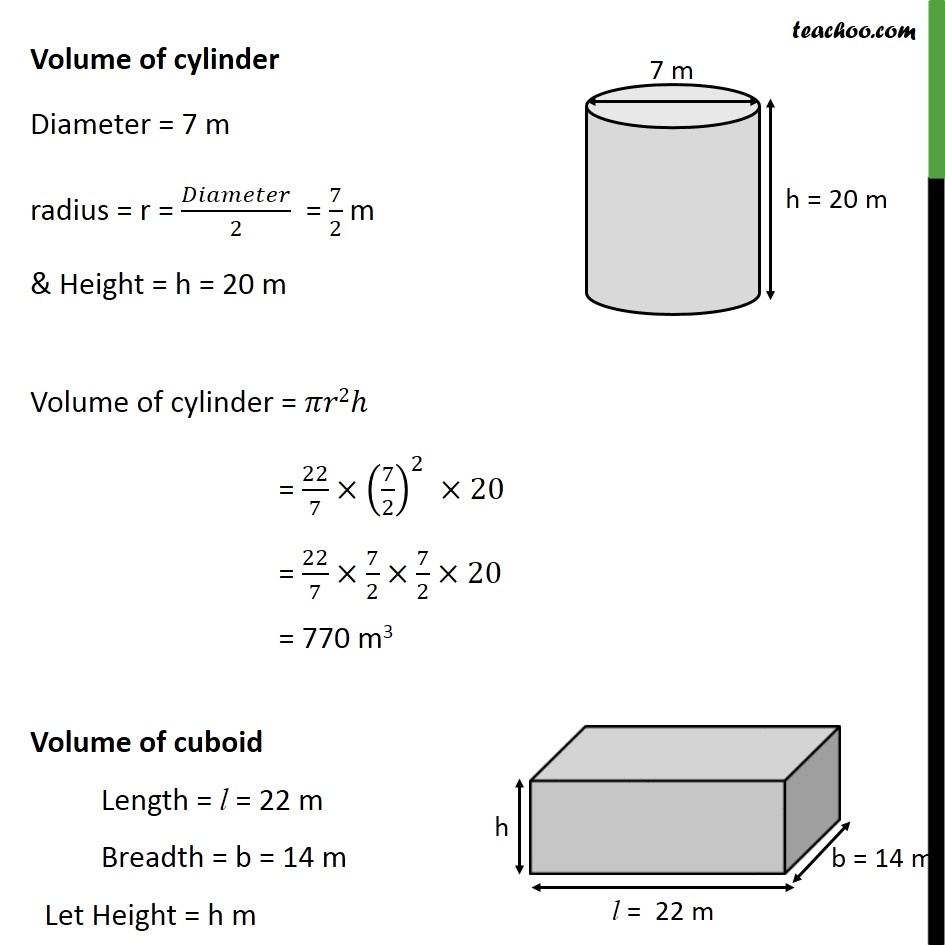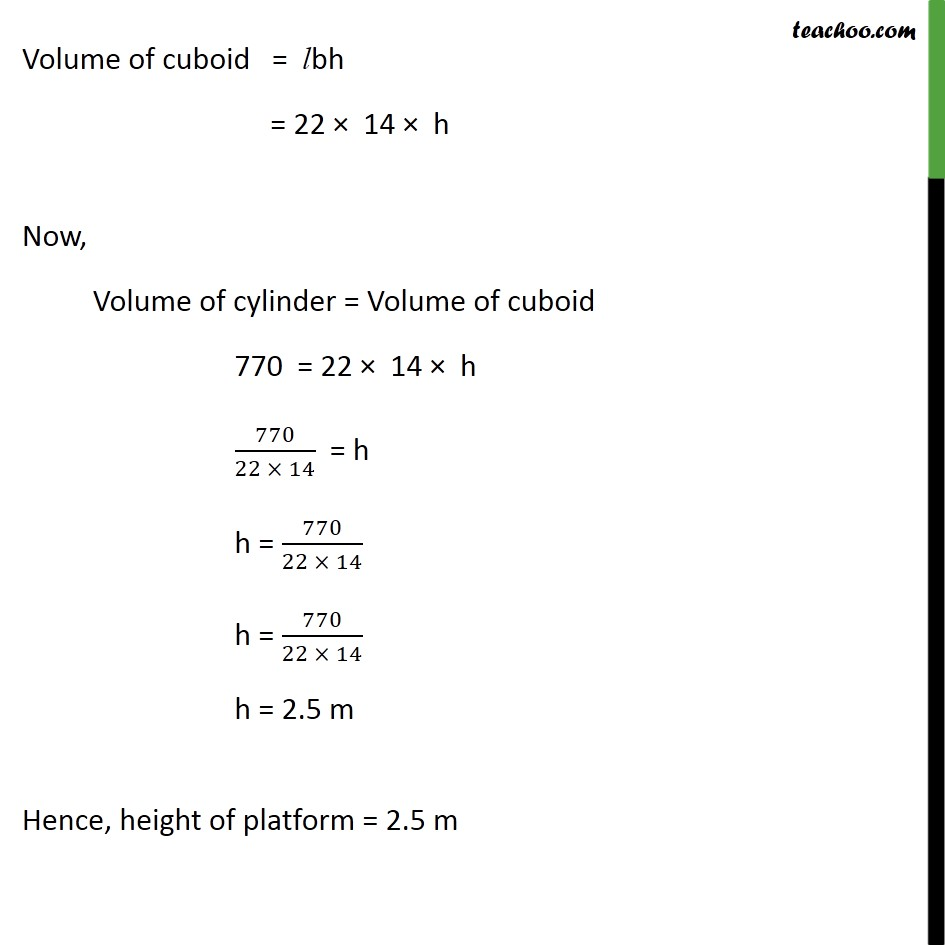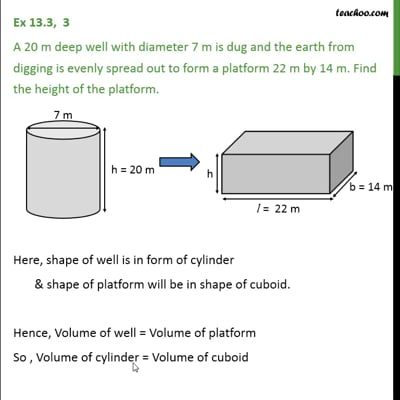Ex 13.3

Chapter 13 Class 10 Surface Areas and Volumes
Serial order wiseThis video is only available for Teachoo black users

Get live Maths 1-on-1 Classs - Class 6 to 12

### Transcript

Ex 13.3, 3 A 20 m deep well with diameter 7 m is dug and the earth from digging is evenly spread out to form a platform 22 m by 14 m. Find the height of the platform. Here, shape of well is in form of cylinder & shape of platform will be in shape of cuboid. Hence, Volume of well = Volume of platform So , Volume of cylinder = Volume of cuboid Volume of cylinder Diameter = 7 m radius = r = /2 = 7/2 m & Height = h = 20 m Volume of cylinder = 2 = 22/7 (7/2)^2 20 = 22/7 7/2 7/2 20 = 770 m3 Volume of cuboid Length = l = 22 m Breadth = b = 14 m Let Height = h m Volume of cuboid = lbh = 22 14 h Now, Volume of cylinder = Volume of cuboid 770 = 22 14 h 770/(22 14) = h h = 770/(22 14) h = 770/(22 14) h = 2.5 m Hence, height of platform = 2.5 m